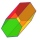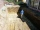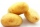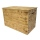# Volume + area of the shape - math problems

#### Number of problems found: 145

• Maximum of volumeThe shell of the cone is formed by winding a circular section with a radius of 1. For what central angle of a given circular section will the volume of the resulting cone be maximum?
• Hexagonal prismCalculate the volume and surface of a regular hexagonal prism with the edge of the base a = 6 cm with the corresponding height v1 = 5.2cm and the height of the prism h = 1 dm.
• Cylindrical tank9.6 hl of water is poured into a cylindrical tank with a bottom diameter of 1.2 m. What height in centimeters does the water reach?We have a regular quadrilateral pyramid with a base edge a = 10 cm and a height v = 7 cm. Calculate 1/base content 2/casing content 3/pyramid surface 4/volume of the pyramid
• Water levelWhat is the area of the water level of the pool, if after filling 25 m3 of water level by 10 cm? a) 25 m2 b) 250 m2 c) 2500 dm2 d) 25,000 cm2
• Hexagonal pyramidCalculate the volume and surface area of a regular hexagonal pyramid with a base edge a = 30 m and a side edge b = 50 m.In a regular quadrilateral pyramid, the height is 6.5 cm and the angle between the base and the side wall is 42°. Calculate the surface area and volume of the body. Round calculations to 1 decimal place.What is the volume of a quadrilateral oblique prism with base edges of length a = 1m, b = 1.1m, c = 1.2m, d = 0.7m, if a side edge of length h = 3.9m has a deviation from the base of 20° 35' and the edges a, b form an angle of 50.5°.A regular quadrilateral pyramid has a volume of 24 dm3 and a base edge a = 4 dm. Calculate: a/height of the pyramid b/sidewall height c/surface of the pyramid
• The regularThe regular triangular prism has a base in the shape of an isosceles triangle with a base of 86 mm and 6.4 cm arms, the height of the prism is 24 cm. Calculate its volume.
• Triangular prismThe triangular prism has a base in the shape of a right triangle, the legs of which is 9 cm and 40 cm long. The height of the prism is 20 cm. What is its volume cm3? And the surface cm2?
• Four prismsQuestion No. 1: The prism has the dimensions a = 2.5 cm, b = 100 mm, c = 12 cm. What is its volume? a) 3000 cm2 b) 300 cm2 c) 3000 cm3 d) 300 cm3 Question No.2: The prism base is a rhombus with a side length of 30 cm and a height of 27 cm. The height of t
• Cylinder containerThe cylindrical container with a diameter of 1.8 m contains 2,000 liters of water. How high does the water reach?
• Hexagonal pyramidFind the volume of a regular hexagonal pyramid, the base edge of which is 12 cm long and the side edge 20 cm.
• The trenchCalculate how many cubic meters of soil needs to be removed from the excavation in the shape of an isosceles trapezoid, the top width is 3 meters, the lower width is 1.8 m, the depth of the excavation is 1 m, and the length is 20 m.
• Alcohol from potatoesIn the distillery, 10 hl of alcohol can make from 8 t of potatoes. The rectangular field with dimensions of 600 m and 200 m had a yield of 20 t of potatoes per hectare. How many square meters of area are potatoes grown to obtain one liter of alcohol?
• Regular hexagonal prismCalculate the volume of a regular hexagonal prism whose body diagonals are 24cm and 25cm long.
• Wooden boxThe block-shaped box was placed on the ground, leaving a rectangular print with 3 m and 2 m. When flipped over to another wall, a print with dimensions of 0.5 m and 3 m remained in the sand. What is the volume of the wooden box?The surface of the regular quadrilateral prism is 8800 cm2, and the base edge is 20 cm long. Calculate the volume of the prismThe water tank has the shape of a sphere with a radius of 2 m. How many liters of water will fit in the tank? How many kilograms of paint do we need to paint the tank, if we paint with 1 kg of paint 10 m2?